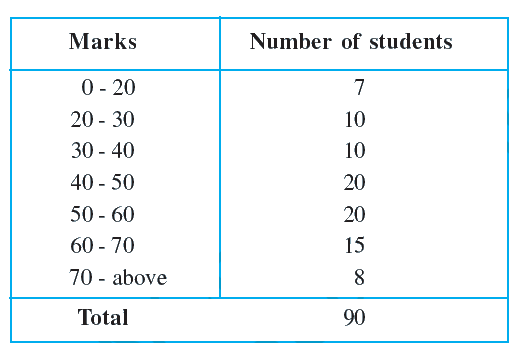# Ex.15.1 Q6 Probability Solution - NCERT Maths Class 9

Go back to  'Ex.15.1'

## Question(i) Find the probability that a student obtained less than $$20\%$$ in the mathematics test.

(ii) Find the probability that a student obtained $$60$$ marks or above.

Video Solution
Probability
Ex exercise-15-1 | Question 6

## Text Solution

What is known?

Marks in different range and respective students.

What is unknown?

Probability of students obtained less than $$20\%$$ in math. Probability of students obtained $$60$$ marks or above.

Reasoning:

The empirical probability $$P(E)$$ of an event $$E$$ happening, is given by:

\begin{align}{P}({E})=\frac{ \begin{pmatrix} \text { Number of trials in which }\\ \text{the event happened } \end{pmatrix} }{ \text { The total number of trials } }\end{align}

Use probability to derive the solution where

Probability (students in range of marks)

\begin{align}=\frac{ \begin{pmatrix} \text { Number of students} \\ \text{ in range of marks } \end{pmatrix}}{\text { Total number of students }} \end{align}

Steps:

Total no of students $$= 90$$

(i)

Probability (a student obtained less than $$20\%$$)

\begin{align} &= \frac{ \begin{pmatrix} \text{No of students who} \\ \text{ obtained less than 20% } \end{pmatrix}}{{{\text{Total number of students}}}} \\ &= \frac{7}{90} \end{align}

(ii)

Probability (a student obtained $$60$$ marks or above)

\begin{align} &= \frac{ \begin{pmatrix}\text{No of students obtained } \\ \text{60 marks or above} \end{pmatrix}}{{{\text{Total number of students}}}} \\ &= \frac{ (15+8)}{90} =\frac{ 23}{90} \end{align}

Learn from the best math teachers and top your exams

• Live one on one classroom and doubt clearing
• Practice worksheets in and after class for conceptual clarity
• Personalized curriculum to keep up with school